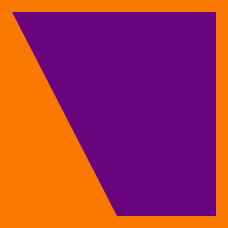Geometry

Challenge Quizzes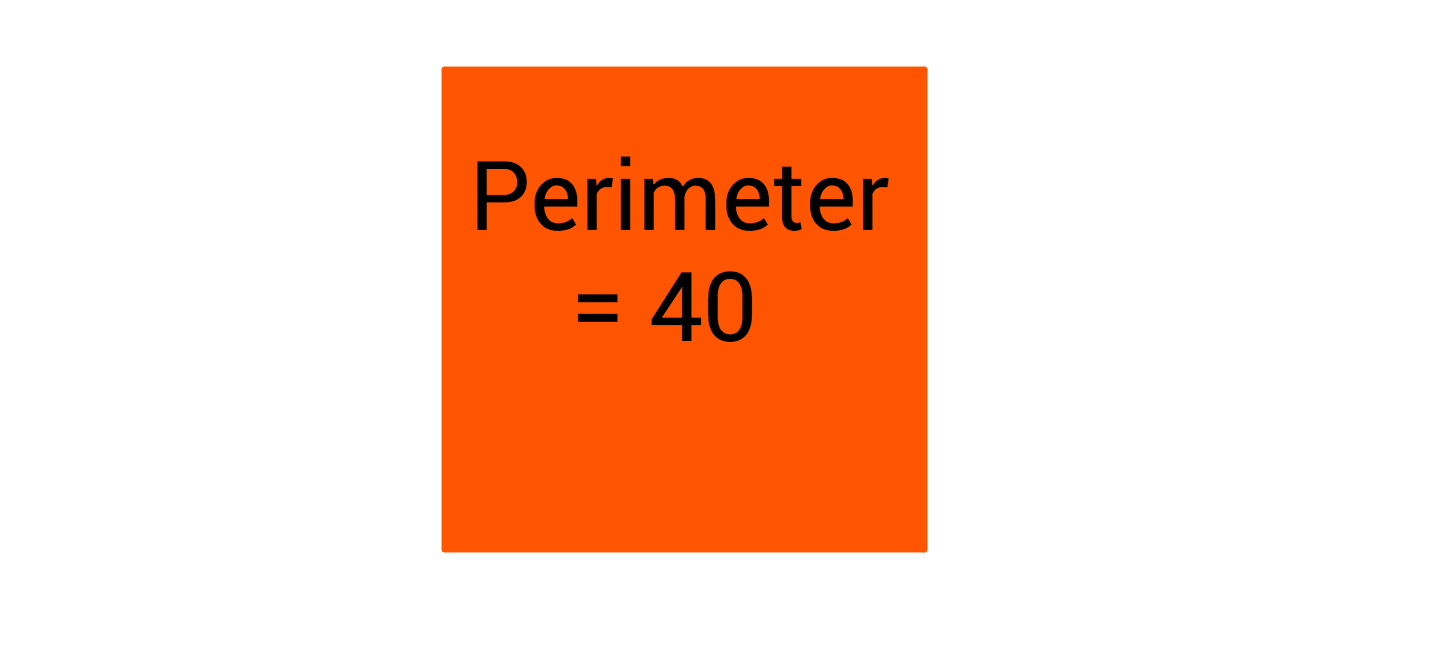The perimeter of a square is 40. What is its area?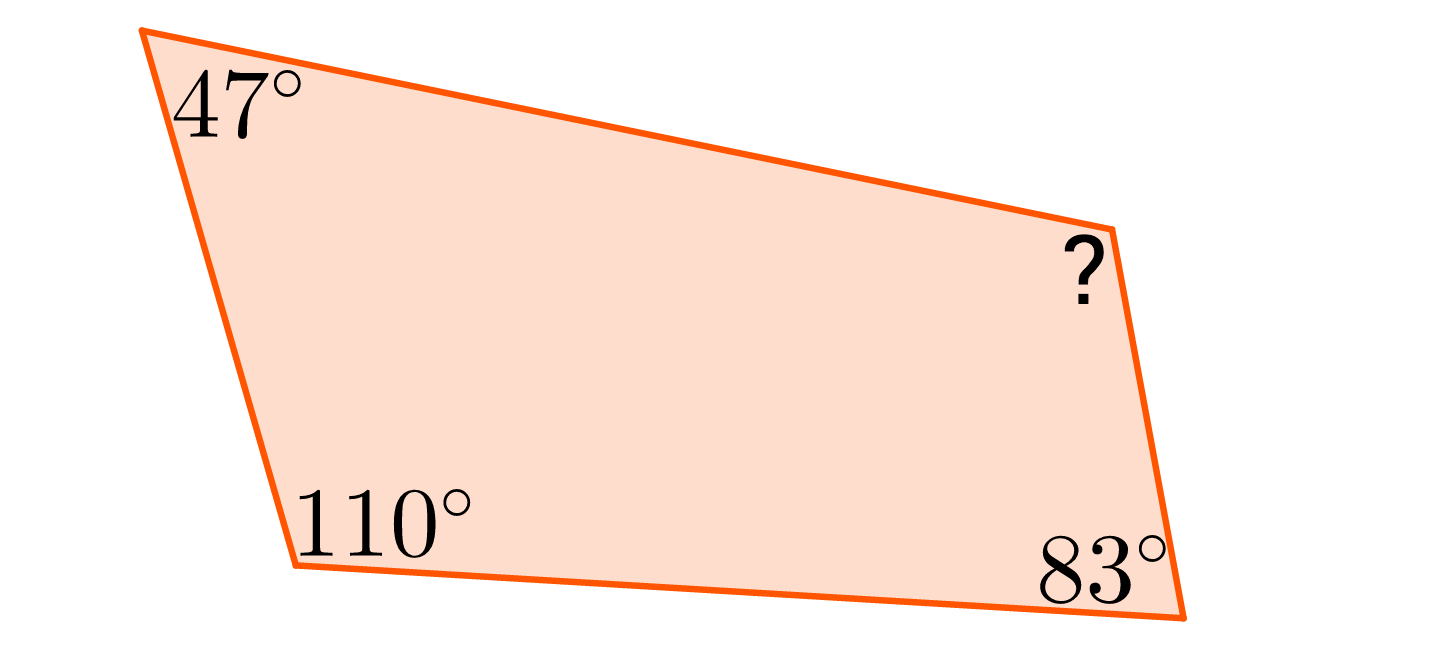Three angles in a quadrilateral measure $110^\circ, 83^\circ, \text { and } 47^\circ.$ What is the measure of the fourth angle?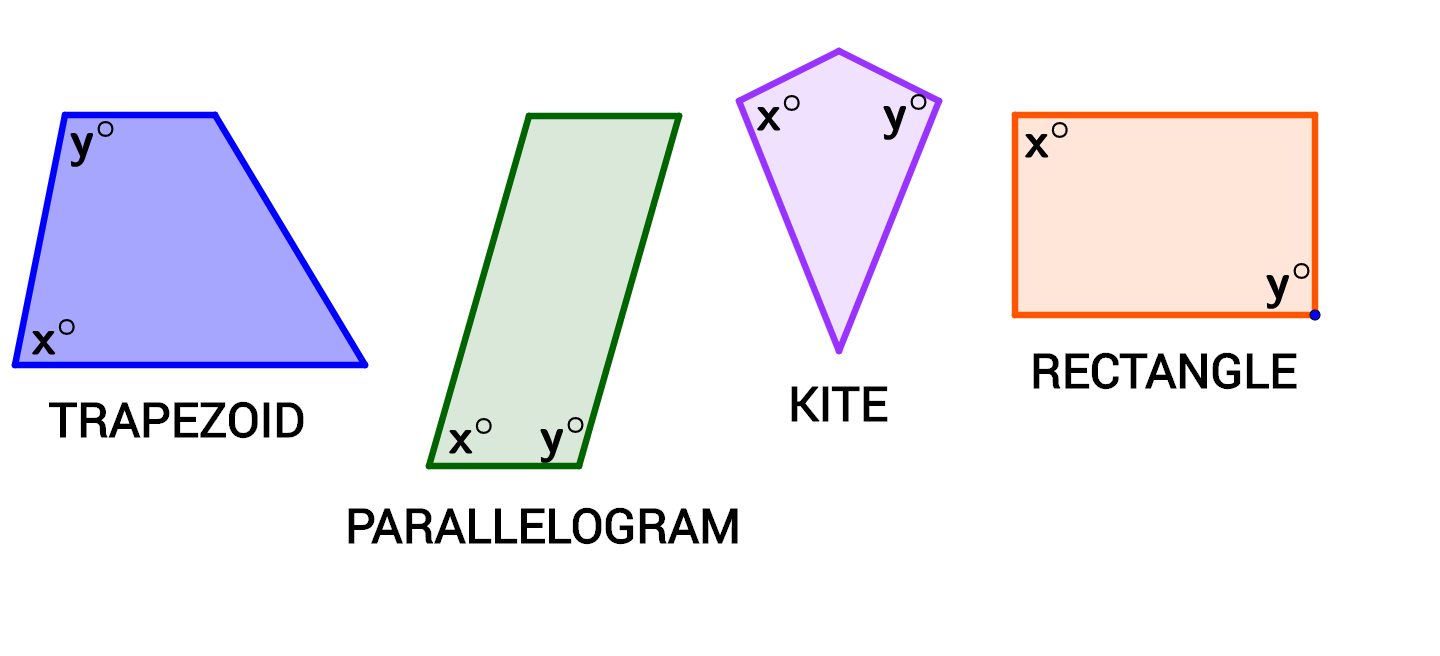For which quadrilateral above does $x + y$ NOT necessarily equal 180?

Which quadrilateral could NOT have one side that is exactly twice as long as another side?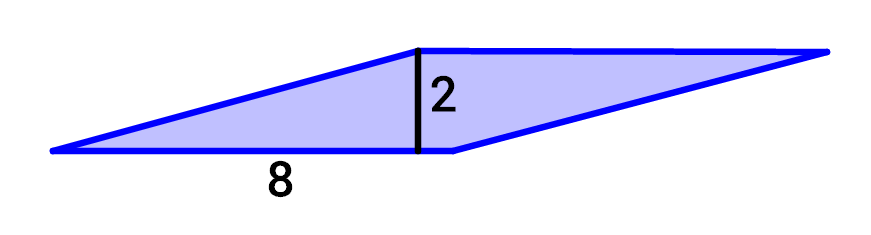A rhombus with a base of 8 and height of 2 will have the same area as a square with a height of __?

×